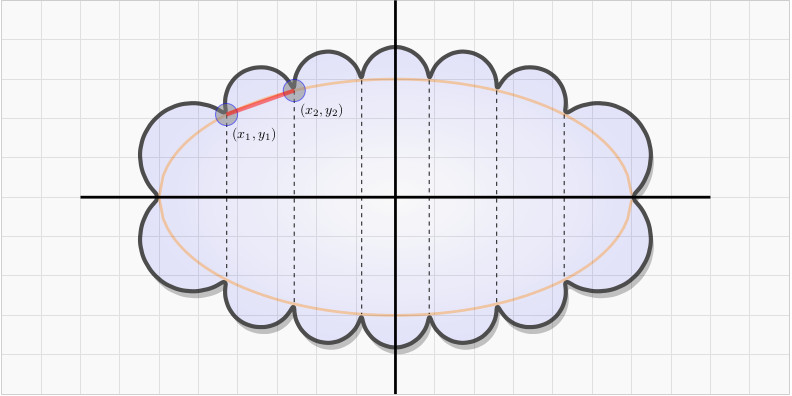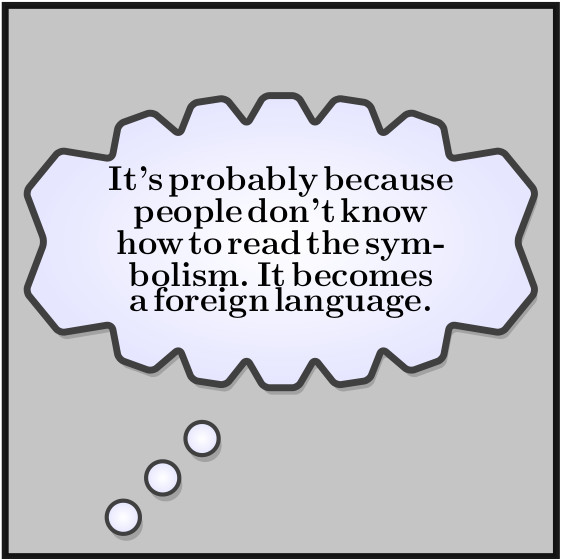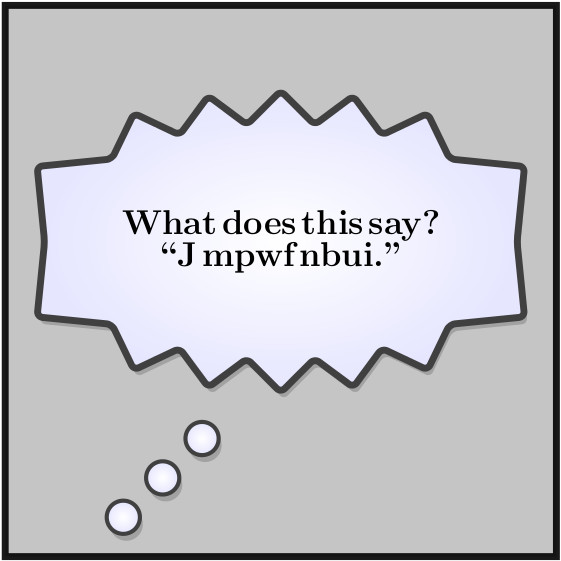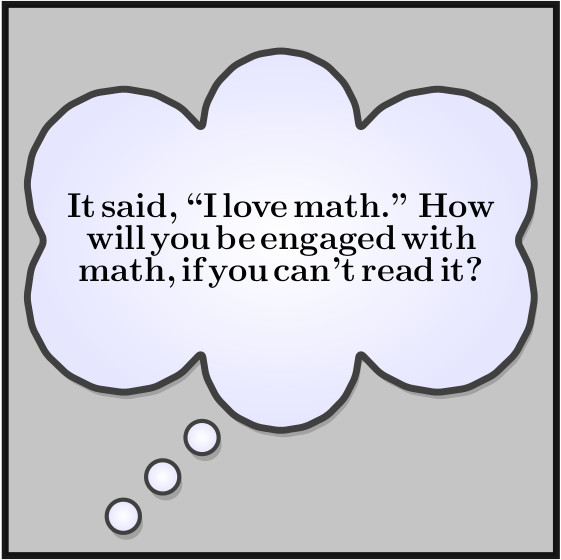# Cartoon Thought Bubbles

In the last article, I discussed how I was able to programmatically generate voice bubbles / balloons to convey what a character or object is saying.

In this article, I’ll show how I create thought bubbles of various forms. Thought bubbles are typically used when a character or object is thinking something, but not saying it out loud.

A thought bubble can look something like thisThe three smaller circles at the bottom left are the thought bubble version of the protrusion we’d see in a voice bubble. Those circles are just “pointing” to the character or object from where the thought is coming. The meat of the thought bubble is where the text is written.

We can see that there is a oval shape to the thought bubble and that the edges look semi-circular. The oval shape can be modeled as an ellipse that’s short and fat. Our canonical formula for an ellipse centered at the origin is $$\frac{x^{2}}{a^{2}} + \frac{y^{2}}{b^{2}} = 1$$ with $$a > b$$.

With that said, let’s dissect the construction of the thought bubble. The image below shows how I go about doing this.We see that the way I constructed this thought bubble was to first mark six uniformly spaced sample points along the major axis of the ellipse. The major axis has width $$2a$$, thus all I am doing is dividing the interval $$[-a,a]$$ into seven equal parts with six partitioning points.

I marked two points $$(x_{1},y_{1})$$ and $$(x_{2},y_{2})$$. There is a thick red line that connects these two points. We can compute the length of this line with the Pythagorean theorem $$d = \sqrt{(x_{2}-x_{1})^{2} + (y_{2}-y_{1})^{2}}$$

Why did I compute this? Well, I want to make those semi-circles. In order to do this I need to know the center of the circle and the radius. The length of the thick red line gives me the diameter $$d$$. Thus, the radius is $$r = \frac{d}{2}$$.

Now where is the center? The center of the circle is just the midpoint of the thick red line. The midpoint is $$(h,k) = \Big(\frac{x_{1} + x_{2}}{2},\frac{y_{1} + y_{2}}{2}\Big)$$

And here is the “tricky” part. How do I get a computer program to draw just the top half of a circle beginning at $$(x_{1}, y_{1})$$ and ending at $$(x_{2},y_{2})$$? There are several ways, but we have to be careful!

Recall that the equation of a circle with center $$(h,k)$$ and radius $$r$$ is $$(x-h)^{2} + (y-k)^{2} = r^{2}$$

So, if I were to sample the circle uniformly along the thick red line, I could, in theory, draw a circle. But I find this to be a bit messy.

Instead we can recognize that I am slicing the circle into half at an angle $$\theta = \arctan\Big(\frac{y_{2}-y_{1}}{x_{2}-x{1}}\Big)$$ Notice how the argument for arctangent is the slope of the thick red line?

Now that I know $$\theta$$, I can sample the circle with radius $$r$$ from $$[\theta, \theta + \pi]$$ and extract $$x$$ and $$y$$ via polar coordinates (note $$\theta \leq \alpha \leq \theta + \pi$$) \begin{align} x & = r\cos(\alpha)\\ y & = r\sin(\alpha)\end{align}

How many times I sample each circle defines how “circular” the circles look.

You will notice that the circles at the far side of the ellipse are larger than the “interior” circles. This happened because I was sampling the ellipse uniformly over the major axis. I was not sampling the ellipse uniformly over the curve. There’s a difference here. In other words, i didn’t sample the ellipse, elliptically.

In any case, once we know how to draw one circle on an ellipse, we can draw as many as we want. I had to write a bunch of functions to handle the individual pieces and then I created one wrapper function called do that when passed the number of circles, number of sampling points per circle, the center of the ellipse, and the height and width of the ellipse, it would return the appropriate $$\LaTeX$$ to render the thought bubble. Thus, do is called do(ncircles,npointsforcircles,h,k,a,b)

Here are a few examples.